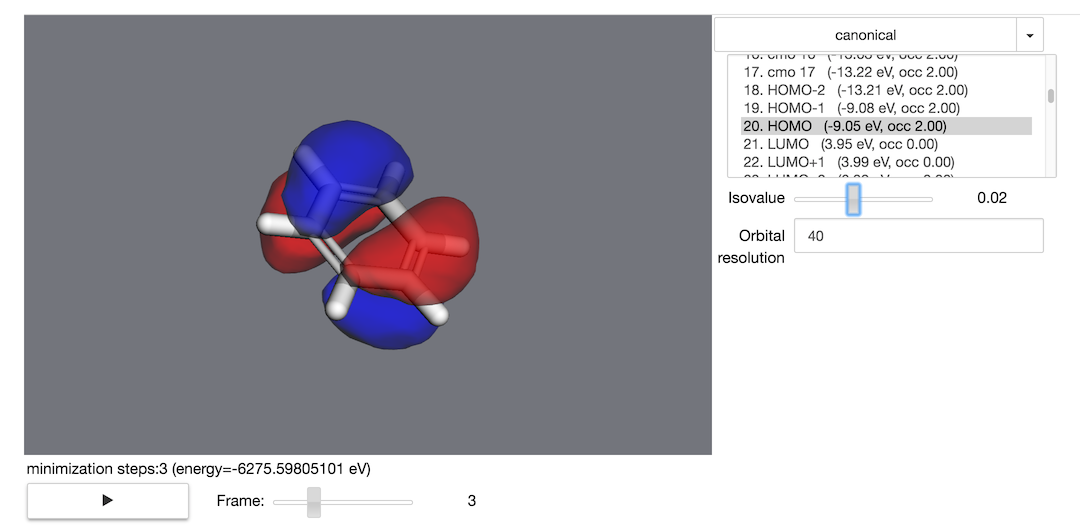# 5. Property calculations¶

## 5.1. Optimize a geometry¶

First, you’ll need to create an EnergyModel for your molecule. Energy models can include quantum chemical, molecular mechanics, or wide assortment of other methods.

We’ll follow the same setup as the previous step:

>>> mol = mdt.from_name('benzene')
>>> mol.set_energy_model(mdt.models.RHF(basis='sto-3g'))


Now that our molecule has an EnergyModel, we can calculate energies and forces:

>>> mol.calculate_forces() # returns vector of forces
>>> mol.calculate_potential_energy() # returns the molecule's energy


You’re now ready to use the molecule’s minimize method:

>>> trajectory = mol.minimize(nsteps=10, frame_interval=1)


And you can visualize the minimization process using the trajectory object:

>>> trajectory.draw_orbitals()